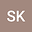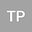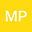Evaluating Catchment Models as Multiple Working Hypotheses: on the Role of Error Metrics, Parameter Sampling, Model Structure, and Data Information Content
•••• +1## Abstract

To evaluate models as hypotheses, we developed the method of Flux Mapping to construct a hypothesis space based on dominant runoff generating mechanisms. Acceptable model runs, defined as total simulated flow with similar (and minimal) model error, are mapped to the hypothesis space given their simulated runoff components. In each modeling case, the hypothesis space is the result of an interplay of factors: model structure and parameterization, choice of error metric, and data information content. The aim of this study is to disentangle the role of each factor in model evaluation. We used two model structures (SACRAMENTO and SIMHYD), two parameter sampling approaches (Latin Hypercube Sampling of the parameter space and guided-search of the solution space), three widely used error metrics (Nash-Sutcliffe Efficiency – NSE, Kling-Gupta Efficiency skill score – KGEss, and Willmott’s refined Index of Agreement – WIA), and hydrological data from a large sample of Australian catchments. First, we characterized how the three error metrics behave under different error types and magnitudes independent of any modeling. We then conducted a series of controlled experiments to unpack the role of each factor in runoff generation hypotheses. We show that KGEss is a more reliable metric compared to NSE and WIA for model evaluation. We further demonstrate that only changing the error metric — while other factors remain constant — can change the model solution space and hence vary model performance, parameter sampling sufficiency, and/or the flux map. We show how unreliable error metrics and insufficient parameter sampling impair model-based inferences, particularly runoff generation hypotheses.